# Draw Seychelles Flag using MATLAB

A colored image can be represented as a 3 order matrix. The first order is for the rows, the second order is for the columns and the third order is for specifying the color of the corresponding pixel. Here we use the RGB color format, so the third order will take 3 values of Red, Green and Blue respectively. The values of the rows and columns depending on the size of the image.

Prerequisite: RGB image representation

Approach:

• Make a 3 order zero matrix of dimensions 300X600X3. 300 denotes the number of pixels for rows, 600 denotes number of pixels for columns and 3 denotes the color coding in RGB format.
• Color the whole image blue by accessing every pixel and changing its RGB value. The RGB color code of blue in Seychelles flag is (0, 63, 135).

After this step the image will look like: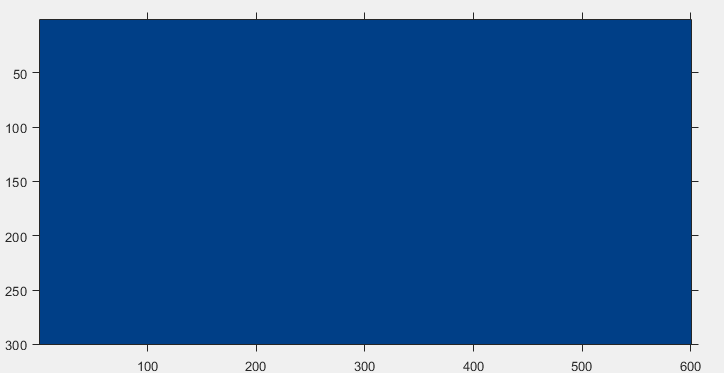• The yellow portion of the flag makes an angle of 57 degrees with the X-axis, therefore its slope will be 1.55. Access each pixel of the image and check whether the slope of the pixel with the origin is less than or equal to 1.55, if true then change its RGB value to that of yellow. The RGB color code of yellow in Seychelles flag is (252, 216, 86).

After this step the image will look like: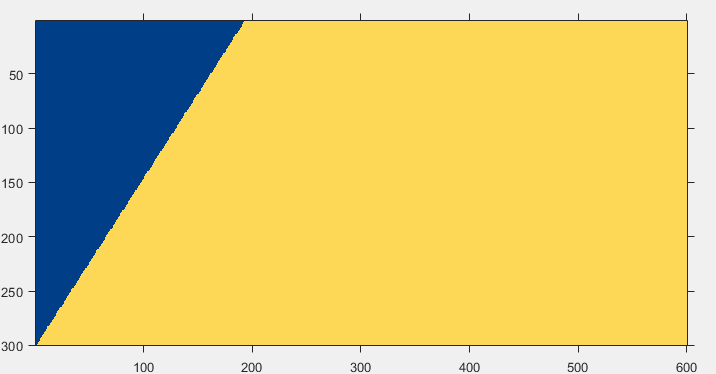• The red portion of the flag makes an angle of 37 degrees with the X-axis, therefore its slope will be 0.75. Access each pixel of the image and check whether the slope of the pixel with the origin is less than or equal to 0.75 if true then change its RGB value to that of red. The RGB color code of red in the Seychelles flag is (214, 40, 40).

After this step the image will look like :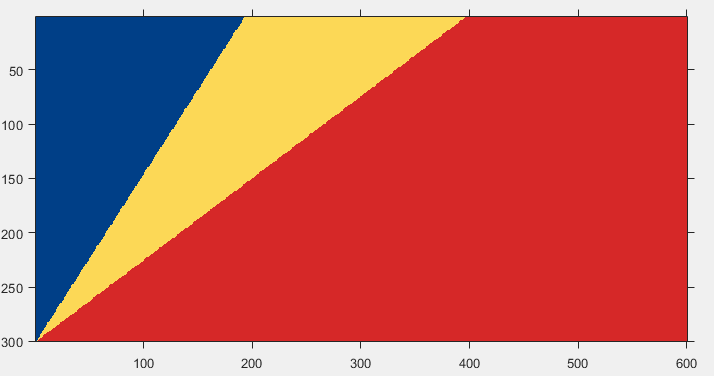• The white portion of the flag makes an angle of 20 degrees with the X-axis, therefore its slope will be 0.36. Access each pixel of the image and check whether the slope of the pixel with the origin is less than or equal to 0.36, if true then change its RGB value to that of white. The RGB color code of white is (255, 255, 255).

After this step the image will look like: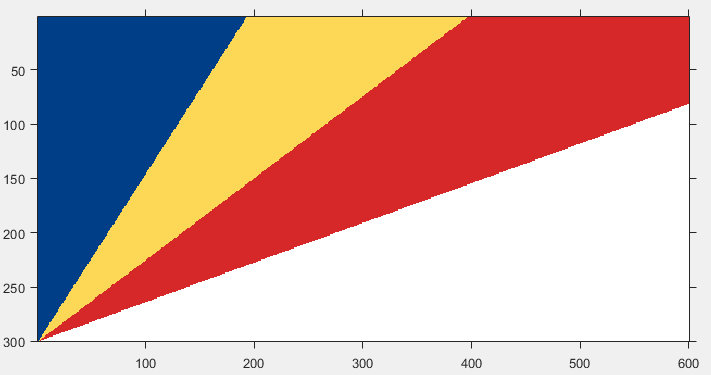• The green portion of the flag makes an angle of 9 degrees with the X-axis, therefore its slope will be 0.15. Access each pixel of the image and check whether the slope of the pixel with the origin is less than or equal to 0.15, if true then change its RGB value to that of green. The RGB color code of green in Seychelles flag is (0, 122, 61).

After this step the image will look like: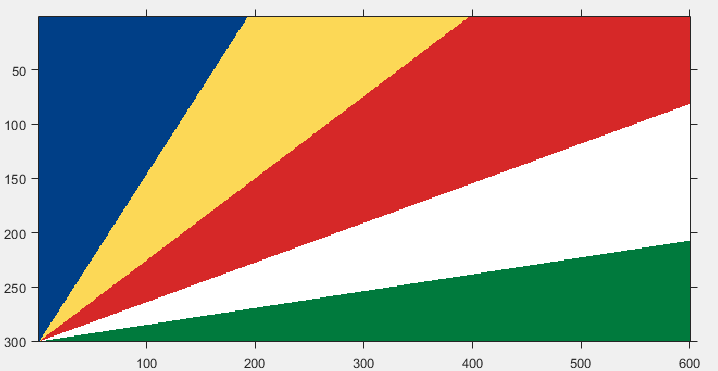Implementation:

 `% MATLAB code to draw Seychelles flag ` ` `  `% initialising a zero matrix of 300X600X3  ` `im=uint8(zeros(300, 600, 3))+255; ` ` `  `% blue ` `for` `i = 1:300 ` `    ``for` `j = 1:600 ` `        ``im(i, j, 1) = 0; ` `        ``im(i, j, 2) = 63; ` `        ``im(i, j, 3) = 135; ` `    ``end`  `end` ` `  `% yellow ` `for` `i = 1 : 300 ` `    ``for` `j = 1 : 600 ` `        ``m = round(abs((i-300)/(j-1)), 2); ` `        ``if` `m <= 1.55 ` `            ``im(i, j, 1) = 252; ` `            ``im(i, j, 2) = 216; ` `            ``im(i, j, 3) = 86; ` `        ``end` `    ``end`  `end` ` `  `% red ` `for` `i = 1 : 300 ` `    ``for` `j = 1 : 600 ` `        ``m = round(abs((i-300)/(j-1)), 2); ` `        ``if` `m <= 0.75 ` `            ``im(i, j, 1) = 214; ` `            ``im(i, j, 2) = 40; ` `            ``im(i, j, 3) = 40; ` `        ``end` `    ``end`  `end` ` `  `% white ` `for` `i = 1 : 300 ` `    ``for` `j = 1 : 600 ` `        ``m = round(abs((i-300)/(j-1)), 2); ` `        ``if` `m <= 0.36 ` `            ``im(i, j, 1) = 255; ` `            ``im(i, j, 2) = 255; ` `            ``im(i, j, 3) = 255; ` `        ``end` `    ``end`  `end` ` `  `% green ` `for` `i = 1 : 300 ` `    ``for` `j = 1 : 600 ` `        ``m = round(abs((i-300)/(j-1)), 2); ` `        ``if` `m <= 0.15 ` `            ``im(i, j, 1) = 0; ` `            ``im(i, j, 2) = 122; ` `            ``im(i, j, 3) = 61; ` `        ``end` `    ``end`  `end` ` `  `% displaying the matrix as an image ` `figure, imshow(im); `

Output :My Personal Notes arrow_drop_upCheck out this Author's contributed articles.

If you like GeeksforGeeks and would like to contribute, you can also write an article using contribute.geeksforgeeks.org or mail your article to contribute@geeksforgeeks.org. See your article appearing on the GeeksforGeeks main page and help other Geeks.

Please Improve this article if you find anything incorrect by clicking on the "Improve Article" button below.

Article Tags :

Be the First to upvote.

Please write to us at contribute@geeksforgeeks.org to report any issue with the above content.